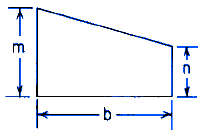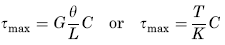Related Resources: calculators

### Trapezoid Torque Deformation Stress Equations and Calculator

Beam Deflection and Stress Equation and Calculators

Torque Applied Deformation and Stress of a Trapezoid Equations and CalculatorALL calculators require a Premium Membership

Preview

Torque Applied Deformation and Stress of a Trapezoid CalculatorWhere:

VL = 0.10504 - 0.10s + 0.0848s2 + 0.06746s3 + 0.0515s4

Vs = 0.10504 + 0.10s + 0.0848s2 + 0.06746s3 + 0.0515s4

s = ( m - n ) / b

Angle of Twist under applied Torque Moment

θ = ( T L ) / ( K G )

Shear Stress MaximumA = ( m + n) b / 2

Where:

θ = angle of twist (radians),
α = degrees,
T = Twisting or torque moment force-length, (in-lbs, N-mm),
L = Length of Section (in, mm),
m, n, b = Length as Indicated (in, mm),
A = area of section (in2, mm2)
τ = Unit shear stress force / area (lbs/in2, N/mm2),
G = Modulus of rigidity force / area (lbs/in2, N/mm2),
K = Polar Moment of Inertia (in4, mm4) for section

Reference:
Roarks Formulas for Stress and Strain, 7th Edition, Table 10.1 Formulas for torsional deformation and stress.